# HBSE 9th Class Science Important Questions Chapter 11 Work and Energy

Haryana State Board HBSE 9th Class Science Important Questions Chapter 11 Work and Energy Important Questions and Answers.

## Haryana Board 9th Class Science Important Questions Chapter 11 Work and Energy

Question 1.
What is work ?
When force is applied on an object and it moves in the direction of force, it is said to be work done.

Question 2.
On which the quantity of work depends ?
The quantity of work depends upon the applied force & distance moved.

Question 3.
What is the formula of work ?
Work = Force x Displacement.

Question 4.
What is the unit of work ?
Newton-metre (Joule).Question 5.
What is energy ?
The capacity of doing work is called energy.

Question 6.
What are the types of mechanical energy ?
Mechanical energy is of two types:
(1) Kinetic energy,
(2) Potential energy.

Question 7.
What is potential energy ?
The energy possessed by a body due to its position or change in shape is called potential energy.

Question 8.
Which type of energies are in aircraft flying on height ?
Both type of energies-kinetic and potential.

Question 9.
Which type of energy is there in a stone lying on a hill ?
Potential energy.Question 10.
What is mechanical Energy ?
The sum of kinetic energy and potential energy of a body is called mechanical energy.

Question 11.
Which type of energy is there when a bullet is fired from a gun ?
Kinetic energy.

Question 12.
Write the formula for kinetic energy.
Kinetic energy = $$\frac {1}{2}$$ mv2, where m → mass, v → velocity.

Question 13.
Write the formula for potential energy.
Potential energy = mgh, where m → mass, g → acceleration due to gravity and h → height.

Question 14.
What is the law of conservation of energy ?
According to this law, energy can neither be created nor be destroyed but can only be transferred from one form to another.

Question 15.
What is the effect on energy when work is done ?
When work is done, energy decrease in some extent.

Question 16.
What is the work done by a man when he lifts a luggage of mass 10 kg on his head and moves 50 metres on horizontal road ?
zero.

Question 17.
From where, our body gets energy ?
From food taken by us.

Question 18.
Which type of energy is used by plants ?
Solar energy.

Question 19.
Write an important use of wind energy.
Wind energy is used to grind the flour by wind mills.

Question 20.
Write the name of any two natural sources of energy.
(i) Sun
(ii) Water.Question 21.
Write any four types of energy.
(i) Mechanical Energy
(ii) Chemical Energy
(iii) Light Energy
(iv) Sound Energy.

Question 22.
What is gravitational potential energy ?
The energy stored in a body when work is done against force of gravity is called gravitational potential energy.

Question 23.
What is mean by elastic potential energy ?
The energy possessed by an object due to change in its shape is called elastic potential energy.

Question 24.
Why spark is produces when two stones collides with each other ?
Because kinetic energy of stone is converted into light energy.

Question 25.
How arrow gets its kinetic energy ?
The arrow converts the potential energy from bow into kinetic energy.

Question 26.
Out of energy and acceleration, which is vector ?
Acceleration is a vector quantity.

Question 27.
The kinetic energy goes on decreasing when it is thrown vertically upward. What will be the motion at highest point ?
Zero.

Question 28.
Write the name of that device which converts electric energy into mechanical energy.
Electric motor.

Question 29.
Which energy is converted in other in a hydroelectric power centre ?
The kinetic energy of water is converted into electric energy.Question 30.
What is power ? What is its unit ?
The rate of doing work is power. Its unit is watt.

Question 31.
If the velocity of an object is increased three times, what will be its kinetic energy ?
The kinetic energy will increase nine times.

Question 32.
Which type of energy is there in a expanded spring ?
Potential energy.

Question 33.
Which type of energy is there in a dam ?
Potential energy.

Question 34.
Which type of energy is there in the spring of watch ?
Potential energy which gives the kinetic energy to the needles of watch. ,

Question 35.
Which type of energy is there in running train ?
Kinetic energy.

Question 36.
What will be potential energy of that body whose height is doubled ?
Its potential energy will be doubled.

Question 37.
What is the relation between 1 kilowatt and watt ?
1 kilowatt = 1000 watt.

Question 38.
Why the palms become hot when rubbed ?
Because kinetic energy of palms is converted into heat energy.

Question 39.
How much energy is required to lift a stone of mass 1 kg to a height of 1 metre ?
9.8 Joules [∵ W = mgh = 1 x 9.8 x 1 = 9.8 joules]Question 40.
A person and his son are running with uniform velocity. If the mass of person become two times more than of his son, then what will be the ratio between kinetic energy of both ?
2 : 1 [ ∵ K.E. = $$\frac {1}{2}$$ mv2 and v is equal]

Question 41.
Which type of energy is required to move for a sailboat ?
The sailboat moves by the kinetic energy of wind.

Question 42.
What will be the energy a body has a capacity to do a work of 10 joules ?
10 Joules.

Question 43.
Write the name of two units of power used in daily life.
Kilowatt and megawatt.

Question 44.
How many watts are there in one horse power ?
1 horse power = 746 watt.Question 45.
What is meant by 1 kilowatt hour energy ?
The energy consumed by a source of 1 kilowatt power in 1 hour.

Question 46.
What is the relation between kilowatt hour and joule ?
1 kilowatt hour = 36,00,000 joules.

Question 47.
Which type of energy is used when a person climbs stairs ?
Muscular energy.

Question 48.
Which type of energy is there in our body ?
Chemical energy.

Question 49.
The person requires the least amount of energy in which of the following activity ?
(i) In swimming
(ii) In movement
(iii) In sleeping
(iv) In running foot.
(iii) In sleeping.

Question 50.
What is simple pendulum ?
Simple pendulum is a metalic bob which is hang by weightless string.

Question 51.
Write the name of energy when simple pendulum is at its extreme point.
Potential energy.

Question 52.
Write the name of energy when pendulum is in middle position.
Kinetic energy.

Question 1.
What is meant by work ? How is it measured ? What is its unit ?
Work done on an object is defined as the magnitude of the force multiplied by the distance moved by the object in the direction of the
applied force.
Thus, Work done = Force x The distance moved in the direction of force
W = F x s
Work is a vector quantity. Unit of work is newton-metre or joule (J).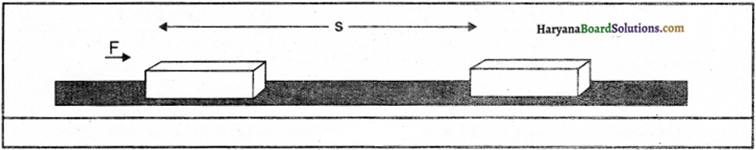Question 2.
What is energy ? Write its unit and definition. What are its various types ?
The capacity of doing work is energy. Its unit is also joule.
Joule: Work done is said to be one joule when a force of 1 newton acting on an object and the object is displaced through 1 m in the direction of force.

Various types of energy:
(i) Mechanical energy
(ii) Heat energy
(iii) Chemical energy
(iv) Electric energy
(v) Nuclear energy’
(vi) Light energy
(vii) Atomic energy
(viii) Magnetic energy
(ix) Sound energy,
(x) Solar energy.Question 3.
Justify with example that moving bodies have capacity to do work ?
The examples are following :
(i) A moving marble has a capacity to displace that marble which is in rest.
(ii) The blowing wind works by rotating the wheels of windmill.
(iii) The moving water also takes the objects from one place to another.
(iv) The blowing wind is helpful for sailing boat.
Thus, we can say that moving bodies have capacity to do work.

Question 4.
What is meant by potential energy and kinetic energy ? Give two examples for each.
Potential energy’: The energy possessed by a body due to its change in position or shape is called the potential energy.
Examples:
(1) The book lying on the table has potential energy due to its position.
(2) A binded watch has potential energy.

Kinetic energy:
The energy possessed by a body due to its motion is called the kinetic energy.

Examples:
(1) Moving air has kinetic energy.
(2) The running water has kinetic energy.Question 5.
Is there energy in following substances ? If yes, then what is the type-potential energy or kinetic energy or both ?
(i) The switched off ceiling fan.
(ii) A man climbing up mountain.
(iii) Flying bird.
(iv) The water in a dam.
(v) The stretched bow more than its shape.
(vi) The rubber locket on a table.
(vii) Stretched locket of rubber.
Yes, all substances have energy’.
(i) A switched off ceiling fan has potential energy only.
(ii) A man climbing up mountain has both potential and kinetic energy.
(iii) A flying bird has both kinetic and potential energy.
(iv) The water in the dam has potential energy.
(v) A stretched bow has potential energy.
(vi) The rubber locket on the table has potential energy.
(vii) Stretched locket of rubber has potential energy.

Question 6.
Write the difference between potential energy and kinetic energy.
The differences between potential energy and kinetic energy are as follows:
Potential Energy (P.E.):
1. The energy possessed by an object due to its position is called potential energy.
2. Potential energy = mgh
3. The potential energy of an object depends upon the height or depth from the surface of earth.

Kinetic Energy (K.E.):
1. The energy possessed by an object due to its motion is called kinetic energy.
2. Kinetic energy = $$\frac {1}{2}$$ mv2
3. Kinetic energy depends upon the motion of the object.

Question 7.
Which type of energy is in following ?
(1) Coiled spring of watch.
(2) Running buffallo.
(3) The arrow released from bow.
(4) A table lying on roof.
(5) Compressed air.
(6) A mango on tree.
(1) Potential energy
(2) Kinetic energy
(3) Kinetic energy
(4) Potential energy
(5) Potential energy
(6) Potential energy.Question 8.
When the capacity of doing work by human body decreases ?
The capacity of doing work by human body decreases in following circumstances :
(1) When a person is ill.
(2) When a person grows old.
(3) When a person takes food less than required, his muscular energy decreases.

Question 9.
What is the relation between work and energy ? Write their units.
The capacity of doing work is called energy. The magnitude of energy is always equal to that work which has a capacity to do that. Energy is measured in joule and the unit of work is also joule.

For example:
To lift a piece of stone of mass 1 kg upto a height of 1 metre requires 9.8 joules energy. Opposite to it, when stone is dropped from one metre, the stone does the same amount of work (9.8 J). Thus, if an object has an energy of 100 joules. It has a capacity of doing work of 100 joules.

Question 10.
How the energy is transferred in key toy ?
There is a spring in key toy or key watch which contracts while binding and stored potential energy in it. After some moments, the spring loses slowly and the toy comes in motion. Thus, potential energy is transferred in kinetic energy.

Question 11.
A ball is thrown upward and it returns back. What is the change in kinetic and potential energy of the ball ? Explain.
When a ball is thrown upward its kinetic energy changes continuously into potential energy. At highest point its whole kinetic energy changes into potential energy. When the ball returns back its potential energy changes continuously into kinetic energy and whole of its potential energy changes into kinetic energy near the earth but on reaching the earth, whole of its kinetic energy changes into potential energy.

Question 12.
A boy is standing by holding some weight in his expanded hand. In this stage, there seems no change in the position of both boy and weight. Is he doing any work ? If yes, then justify.
Yet, there is no change in the position of boy and weight externally, but internally the boy is working. The muscles of boy are stretched due to weight on palm. Thus, their shape get changes. The heart pumps more blood into muscles. The energy of boy wastes in all these chemical activities and he tired soon. If the boy stand more enough in same position, the external change will be observed as sweating.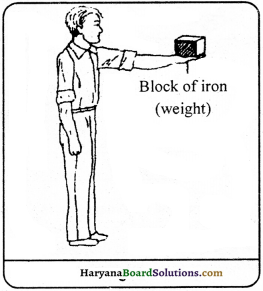Question 13.
How much work is done when an artificial satellite revolve round the earth ? Explain in brief. –
The artificial satellite revolves round the earth in almost circular track. The gravitational force between the earth and the satellite works along the line joining the earth and satellite and the satallite moves perpendicular to the direction of force of gravity. Thus, the magnitude of displacement of satellite in the direction of force will be zero and the work done by the earth on the satellite will also be zero.Question 14.
How the kinetic energy of wind is utilised ? Write its important uses.
The electric energy can be produced by the energy in the blowing air. It has much waste. Thus, wind mill is used in grinding flour and drawing out water from ponds. A small amount of electric energy is produced from wind mill. Those places where the wind blows throughout the years, wind mill are used to grind flour and drawing out water.
Important uses of mechanical energy of wind :
(1) The fields can be irrigated by drawing water from ponds.
(2) It can be used in grinding the flour.
(3) The mechanical energy of wind can be converted into electric energy by joining the various wind wheels together.

Question 15.
What is water energy ? Write its two important uses.
The running water has a capacity to do work due to its kinetic energy, which is known as water energy.

Uses of water energy: Following are the uses of water energy :
(1) Water energy is converted into electric energy and it is used in houses and factories.
(2) Water energy is used to grind flour by windmills.
(3) The heavier wooden pieces is taken from one place to another due to water energy.

Question 16.
How water energy is used to produce electricity ?
The kinetic energy of running water is stored as potential energy in dam. The stored water is dropped on the larger blades of water turbines. The shaft attached with running turbine rotates the generator or dyanmo quickly. The rotating coil of dyanmo produces electricity which is called ‘hydroelectric power’. The electricity is produced on Bhakhra dam by this method. This is also known as the principle of hydroelectricity.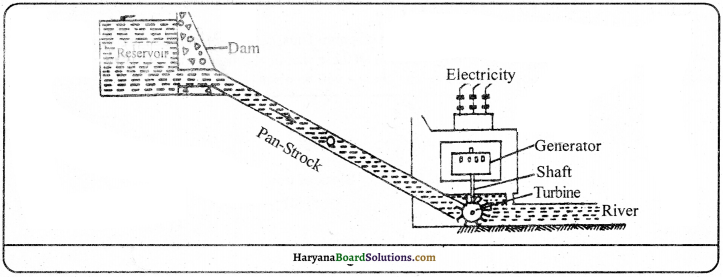Question 17.
Why the nail becomes hot when it is hammered in the sheet of wood ?
The nail becomes hot when it is hammered in the sheet of wood because the lifted hammer has potential energy due to its position. When the hammer is dropped on the nail, it comes in rest position, but transfers, its whole energy in the nail. As a result of this, the nail gets some kinetic energy and moves inside the wood. When the nail enters completely and we continuous hammering the nail becomes hot because the mechanical energy of the hammer is transferred in nail and changes into heat energy.

Question 18.
What are the benefits of the solar energy ?
The benefits of the solar energy are as follows :
(1) The solar energy is used in solar cooker to prepare food,
(2) Solar energy is used to warm the water by solar heater in hotels, hospitals and industries.
(3) Solar energy is used in the production of electricity.
(4) It is used to melt the metals in solar furnances.

Question 19.
Differentiate among work, energy and power. Write the SI unit for each. Or What is Power? Write its S.I. unit.
Work:
When force is applied on a body and it moves in the direction of force, it is said to be work done.
Thus, Work (W) = Force (F) x Distance (S)
SI unit of work is joule.

Energy: t he capacity ot doing work is called energy. SI unit of energy is joule.

Power: The rate of doing work is called power.
Thus,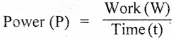SI unit of power is watt.

Question 20.
What do you mean by transfer of energy ? Write two examples of transfer of energy.
According to the law of conservation of the energy, The energy can neither be created nor destroyed. It can be transferred from one form to another. It is called the transfer of energy. Thus, the total magnitude of energy is never changed.

Examples:
(1) Ice on mountains melts and becomes water. This is the transfer of potential energy into kinetic energy.
(2) The electric energy in bulb changes into light energy and glows.Numerical Questions

Question 1.
A boy of mass 40 kg is running with a ve’ocity of 0.5 ms. What is his kinetic energy ?
Solution:
Here,
m = 40 k
v = 0.5 ms-1
We know that,
Kinetic Energy (K.E.) = $$\frac {1}{2}$$ mv2
= $$\frac {1}{2}$$ x 40 x 0.5 x 0.5 = 5 Joule Answer:

Question 2.
An object of mass 15 kg is moving with a uniform velocity (if 4 ms. What is tue kincth energy possessed by the object?
Solution:
Here
Mass of the body (m) = 1 5 kg
Velocity of the body (v) = 4 rn/s
We know that
Kinetic Energy (K.E) = $$\frac {1}{2}$$ mv2
= $$\frac {1}{2}$$ x 15 x 4(2)2
= $$\frac {1}{2}$$ x 15 x 16
= 120 Jule

Question 3.
A stone of mass 100 gram is kept at a height ofS metre. What kind of energy does it possess and find out its value.
Solution:
The stone has potential energy because of height.
Here. m = 100g = $$\frac {100}{1000}$$ = 0.1 kg
g = 9.8 ms-2
h = 5m
We know that.
Potential Energy (P.E.) m x g x h = 0.1 x 9.8 x 5 = 4.9 Joule

Question 4.
To what height can an object of mass 1 kg be raised by giving it an energy of 980 joule?
Solution:
Here.
m = 1 kg
g = 9.8 ms-2
h = ?
Potential Energy (RE.) = 980 J
We know that,
Potential Energy (RE.) = m.g.h.
or 980 = 1 x 9.8 x h
or h = $$\frac{980}{1 \times 9.8}$$
= 100 m

Question 5.
What is the increase in kinetic energy of a particle, if its velocity is increased to four times?
Solution:
Suppose the initial velocity of the particle = v
and its final velocity = 4 v
Suppose the mass of the particle = m
∴Initial kinetic energy (K1) of the particle = $$\frac {1}{2}$$ mv2 ………….(i)
Final kinetic energy (K2) of the particle = $$\frac {1}{2}$$m (4v)2
= $$\frac {1}{2}$$m x 16 v2 …………..(ii)
From equations (i) and (ii),
$$\frac{\mathrm{K}_1}{\mathrm{~K}_2}=\frac{\frac{1}{2} \mathrm{mv}^2}{\frac{1}{2} \mathrm{~m} \times 16 \mathrm{v}^2}=\frac{1}{16}$$
or K2 = 16K1
Thus, the increase in kinetic energy is 16 times the initial kinetic energy.

Question 6.
The kinetic energy of a ball of mass loo g is 20 joule. Calculate its velocity.
Solution:
Here,
m = 100 g = $$\frac {100}{1000}$$kg = 0.1 kg
v = ?
Kinetic Energy (K.E.) = 20 J
We know that, Kinetic Energy (K.E) = $$\frac {1}{2}$$ mv2
Or 20 = $$\frac {1}{2}$$ x 0.1 x v2
v2 = $$\frac {20 x 2}{0.1}$$ = 400
or v = $$\sqrt{400}$$ = 20 ms-1

Question 7.
A coolie lifts an object of mass 20 kg from the earth to 1.5 m and keep It on his head. Calculate the amount of work done by him on the object.
Solution:
Here,
Mass of the object (m) = 20kg
Displacement (s) = 1 .5m
Work done (W) = F x s = mg x s
= 2okg x 10 ms-2 x 15 m
= 300kgms-2 m
= 300Nm= 300J
The amount of work done by the coolie on the object is 30 joules.Question 8.
A man of mass 50 kg jumps to a height of 1.2 m. What is its potential energy at the highest point?
Solution:
Here,
m = 50kg
g = 9.8 ms-2
h = 12m
We know that,
Potential Energy (P.E.) m x g x h
= 50 x 9.8 x 1.2
= 588 J
Thus, at the highest point the potential energy of the man is 588 J.

Question 9.
With what velocity should a man of mass 50 kg run so that his kinetic energy becomes equal to 625 J.
SolutIon:
Here,
m = 50kg
v = ?
Kinetic Energy (K.E.) = 625 J
We know that,
Kinetic Energy (K.E) = $$\frac {1}{2}$$ mv2
Or 625 = $$\frac {1}{2}$$ x 50 x v2
v2 = $$\frac{625 \times 2}{50}$$ = 25
or v = $$\sqrt{25}$$ 5ms

Question 10.
A man throws a stone of mass 10 kg from a ladder of height 5m. When ¡t reaches the ground, what will be its kinetic energy ? What will be its velocity at the nearest point to the ground?
Solution:
Here,
m = 10kg
h = 5m
v = ?
Kinetic Energy (K.E.) = ?

We know that,
The kinetic energy (K.E.) of the stone = The loss in potential energy due to falling of the stone
= mgh
= 10 kg x 9.8 ms-2 x 5m = 490J ……………(i)
Because kinetic energy = $$\frac {1}{2}$$ mv2 .(ii)
From eqns. (i) and (ii),
$$\frac {1}{2}$$ mv-2 = 490J
or $$\frac {1}{2}$$ x 10kg x v2 = 490J
or v2 =$$\frac{490 \times 2}{10}=\frac{980}{10}$$ = 98
or v = $$\sqrt{98}$$ = 9.9 ms-1 (approx.)Question 11.
A car of mass 1000 kg is moving with a velocity of 30 ms-1. After applying brakes it stops with uniform acceleration at a distance of 50m. Calculate the force and the work done by the brakes?
Solution:
Here,
m = 1000kg
u = 30 ms-1
v = 0 ms-1
s = 50m
F = ?
W = ?
We know that, v2 – u2 = 2as
a = $$\frac{v^2-u^2}{2 s}=\frac{(0)^2-(30)^2}{2 \times 50}=\frac{-900}{100}$$ ms-2
= – 9 ms-2 So retardation = 9ms-2
F = m.a.l000kg x 9ms2 = 9000 N
The force applied by the brakes on the car (F) = 9000 N
Work done(W) = F x s = 9000 N x 50 m = 450000 J = 450 kJ

Question 12.
A man of mass 50kg climbs a vertical height of 10m on a mountain in 20 seconds. What ¡s his power ? (Given that g 9.8 ms-2)
Solution:
Here,
F = m x g
= 50 x 9.8 = 490N
Now, W = F x S = 490N x 10m = 4,900 Nm
Time (t) = 20 seconds
Power (P) = $$\frac{\text { Work done }}{\text { Time taken }}=\frac{4,900}{20}$$ = 245 watt

Question 13.
A boy of mass 40 kg runs up a staircase of 45 steps in 9 sec. If the height of each step is 15 cm, Calculate his power ? (The value of g is 10 ms2)
Solution:
Weight of the boy (mg) 40kg x 10ms2 = 400N
Total height of the steps (h) = 45 x 15/100m = 675m
Time taken to climb the steps (t) = 9s
We now that
Power (P) = Time taken = $$\frac{\mathrm{mgh}}{t}$$ = $$\frac{400 \mathrm{~N} \times 6.75 \mathrm{~m}}{9 \mathrm{~s}}$$ = 300 W
The power of the boy is 300 W.

Question 14.
A man of mass 50 kg climbs 30 steps in 30 sec. If height of each step is 20 cm. Then calculate the power to climb the total steps.
Solution:
Mass of the man (m) = 50 kg
Total number of steps = 30
height of each step = 20 cm = $$\frac {20}{100}$$ m = 0.2 m
Total height covered by the man = 30 x 0.2 m = 6m
Acceleration due to gravity (g) = 10 ms-2
Thus, work done by the man = m.g.h = 50 x 10 x 6J = 3000J
Time taken to climb the steps = 30 s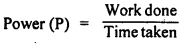= $$\frac{3000 \mathrm{~J}}{30 \mathrm{~s}}$$
= 100 Js-1 = 100 W(watt)Question 15.
An electric bulb of 100 Wis used for 2 hours, how much electrical energy is consumed?
Solution:
Power of the bulb = 100W
Time = 2 hours 2 x 3600 = 7200 sec.
Energy consumed = Power x Time
= 100 x 7200Ws = 720000 joule

Question 16.
A rocket has a mass of 3 x 106 kg. Calculate (a) its potential energy (b) its kinetic energy, at a height of 25 km moving with a velocity of 1 km/s. (Take g = 10 ms-2)
Solution:
Here,
Mass of the rocket (m) 3 x 106 kg
Velocity of the rocket (v) = 1 k ms-1 = 1000 ms-1
Height of the rocket (h) = 25 km = 25000 m
Acceleration due to gravity (g) = 10 ms-2

(a) Potential Energy = m.g.h.
3 x 106 x 10 x 25000 J
= 7.5 x 1011

(b) Kinetic Energy = $$\frac {1}{2}$$ mv2
= $$\frac {1}{2}$$ x 3 x 106 x (1000)2 J
= 7.5 x 1011J

Question 17.
A horse is pulling a cart moving with a velocity of 18 kmh-1 with a force of 300 N. Find out the power of the horse in watt and also in horse power?
Solution:
Here,
Velocity of the cart(v) = 18 kmh-1
= $$\frac{18 \times 1000}{3600}$$ ms-1 = 5 ms-1
The force exerted by the horse on cart (F) = 300 N
m.g. = 300N
Work done = m.g.h
= 300 x 5 = 1500J= $$\frac{15000}{1s}$$ = 1500 w
We know that, 746 W = 1 horsepower
1 waft = $$\frac {1}{746}$$ horsepower
1500 watt = $$\frac {1}{746}$$ x 1500 horsepower
= 2 horsepower

Do Yourself

(1) A car is moving with a uniform velocity of 54 kmh-1 What will be the kinetic energy of a child of mass 40 kg sitting inside it?
[4500J]

(2) Calculate the power of that pump which stores the water of loo kg in 25 seconds into a tank kept at a height of 19m. (Let g = 10 ms2)

(3) What will be the energy of an object of mass 10 kg at a height of 40 m from the ground level?
[Take g 100 ms2] 

(4) A force of 5 N is acting on a body. The body displaces to a distance of 2m in the direction of the force. Find out the work done on the object.
[103)(5) An object of mass 15 kg is moving uniformly with a velocity of 4 ms2. Calculate the kinetic energy of the object.
[120J]

(6) What will be the height from the ground level where an object of mass 1 kg will have a potential energy of 1 joule if g= 10 ms2?
[0.1 m]

(7) If 4900 joule of energy is utilised in lifting a body of mass 50 kg to a certain height, then what will be the height of the object if g = 9.8 ms2?
[10m]

(8) A body of mass 3 kg starts falling down from rest position. After 3 sec., what will be its kinetic energyifgl0m.c2?
[1350m]

(9) An object of mass 10 kg is lifted to a height of 6m from the ground level. Calculate the energy present in the object. The value of g is
9.8 ms2.
[588 J]

(10) An object of mass 12 kg is kept at a certain height. 1f its potential energy is 480 J; calculate its height from the ground level.
[Take g = 10 ms2] [4 m]

(11) A man of mass 50kg takes a packet of mass 20kg upto the terrace of a building of height 14m, what is the work done by the man ?
[Take g = 10 ms2] [9604 J]

(12) A vehicle of mass 1800 kg is moving with a velocity of 40 ms. After applying brakes it stops with a uniform acceleration at a distance of 200 m. Calculate the force applied and work done by the brakes.
[1440 kJ]

(13) What will be the increase in kinetic energy of a particle if its velocity is doubled?
[4 times ]

(14) How much amount of work is done on lifting an object of mass 2 kg to a height of 2m if the value of g is 9.8 ms2?
[39.2J1(15) The height of’Kutubminar’ is 70m. What is the work done by a man of mass 50 kg on reaching the top?
[Take g 9.8 ms-2] [3430011

(16) A stone of mass 10g is kept at a height of 5m. What kind of energy is present in it and calculate its value?
[Take g 9.8 ms-2] [0.49J]

(17) Two girls A and B of mass 400 N each, climbs a rope upto a height of 8m. IfA takes 20 sec. to do this work while B takes 50 sec.,
then calculate the individual power of both the girls.
[A 160 W, B = 64W]

(18) Find the energy in KWh consumed inS hours by four devices of power 500 W each.
[10Kwh]

Essay Type Questions

Question 1.
Define potential energy. Derive an expression for the potential energy of a body.
Potential Energy:
The energy possessed by a body due to its position or change in shape is known as potential energy.

Expression for potential energy:
Consider a body of mass m kept on the.ground level at point ‘A’. it is raised to a point ‘B’ by applying a force F on it. The distance between A and B is ‘h’.
As we know that, the force F acting on the body to lift it, is equal and opposite to the gravitational force
F = $$\frac{G M_e m}{\mathrm{R}_e{ }^2}$$ …………(i)

Where, Me = Mass of the earth
Re = Radius of the earth
But, g = $$\frac{G M_e m}{\mathrm{R}_e{ }^2}$$ (g = acceleration due to gravity) ………..(ii)
From equations (i) and (ii),
F = mg
The work done in raising the body to a height ‘h’
W = F x S (∵ s = h) = mgh
Because work done is same as the energy. Therefore, at a height ‘h’ the potential energy of the object is,
Potential Energy (P.E.) = mgh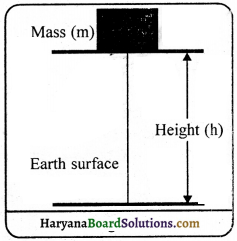Question 2.
Define kinetic energy. Obtain an expression for it.
Kinetic Energy:
The energy possessed by a body due to its motion is called kinetic energy.

Expression:
Let us suppose a body of mass m is dropped from a height h and its velocity becomes V.
on reaching the ground level Then –
Initial velocity of the body (u) = 0
Acceleration (a) = g (acceleration due to gravity)
Final velocity = v
Distance (s) = Height (h)
We know that, v2 – u2 = 2as
v2 – 0 = 2gh
v2 = 2gh
h = $$\frac{v^2}{2 g}$$ …………. (i)
As the object comes down, its potential energy decreases and kinetic energy increases. When the body strikes to the ground level, its hoIe potential energy is converted into kinetic energy. According to the law of conservation of energy, Energy can neither be increased nr be decreased.”
Thus, kinetic energy at ground level = Potential energy at height h.
Kineiic Energy = mgh
or K.E. = mg x $$\frac{v^2}{2 g}$$ (∵ h = $$\frac{v^2}{2 g}$$)
K.E. = $$\frac {1}{2}$$ mv2Question 3.
Define Law of conservation of energy. Give an example of conservation of energy.
Law of conservation of energy-According to this law, energy can neither be created nor be destroyed. ¡t can only be transfèrred from one form to another. When the energy is released in any form, the same amount of energy appears in other forms. Thus, total energy of the universe remains constant. Suppose, an object of mass m starts falling from a height h, on the ground level.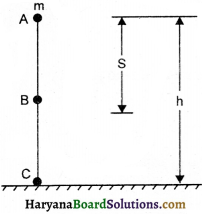(a)EnergyatpointA, PotentialEnergy = mgh
Kinetic Energy = 0
∴ Total energy = mgh + 0 = mgh ……………(i)

(b)Energy at point B:
Suppose the object falls from A to B at a distance s, then its height from ground level will be (h-S).
∴ Potential energy = mg (h-s)
and v2-u2 = 2gs
v2 = 2gs [∵ u = 0]
Kinetic Energy = $$\frac {1}{2}$$ mv2 = $$\frac {1}{2}$$m x 2gs = mgs
Total Energy = mg (h-s) + mgh = mgs – mgs + mgs = mgh …………(2)

(c) Energy at point C; v2-u2 = 2gh
v2 = 2gh [∵u = 0]
Kinetic Energy = $$\frac {1}{2}$$ mv2 = $$\frac {1}{2}$$ m x 2gh = mgh
and Potential Energy = 0
Total Energy = mgh + 0 = mgh ……………(iii)
in this way we observe from eQuestion (i), (ii) and (iii) that the energy of the object remains constant at every point. There is potential energy in the object at highest point. When it falls litle downs same part of potential energy is converted into kinetic energy and on ground level whole potential energy is converted into kinetic energy.

Practical Work

Experiment 1.
Describe by an experiment that the wark done by a force can either be positive or negative.

Procedure:
A child is pulling a toy car parallel to the ground as shown in figure. The child has exerted a force in the direction of displacement of car. In this situation, the work done will be equal to the product of the force and displacement. In this situation the work done by the force is taken as positive. Consider a situation in which an object is being displaced by the action of forces and we identit one of the forces, F acting Opposite to the direction of the displacement s, thus, the angle between the two directions is 1800.In such a situation, the work ‘ done by the force F is taken as negative and denoted by the minus sign. The work done by the force is F x (-s) or (-F x s). When the force acts opposite to the direction of the displacement, the work done is negative. When the force acts in the direction ofdisplacernen, the work done is positive.

Experimenf 2.
Describe by an activity in which both positive and negative forces are working.

Procedure:
When an object is lifted up, both positive and negative forces work, because the force applied by us, displaces the object upward and called positive force, while the force of gravity applied by earth pulls the object downward which is called negative force.Experiment 3.
Describe by an experiment that the object at more height has more energy.

Procedure:
(1) Take a heavy ball. Drop it on a thick bed of sand. A wet bed of sand would be better. Drop the ball on the sand bed from height of about 25 cm. The ball creates a depression.
(2) Repeat this activity from heights of 50 cm, I m and 1.5 m.
(3) Ensure that all the depressions are distinctly visible.
(4) Mark the depressions to indicate the height from which the ball was dropped.
(5) Compare their depths.

We will observe that when the ball was dropped from the height of 1.5 m, the depression was the deepest; which proves that the object at more height has more energy which makes the deepest depression.

Expereiient 4.
Describe by an experiment that the kinetic energy of an object increases by increasing its mass.

Procedure:
(1) Set up the apparatus as shown in figure
(2) Place a wooden block of known mass in front of the trolley at a convenient fixed distance.
(3) Place a known mass on the pan so that the trolley starts moving.
(4) The trolley moves forward and hits the wooden block and displaces the block.
(5) Note down the displacement of the block. It is, clear that the work is done on the block by the trolley as the block has gained energy.
(6) Repeat this activity by increasing the mass on the pan.

You will observe that the displacement will increase with the increase in mass, which proves that the kinetic energy increases by increasing the mass.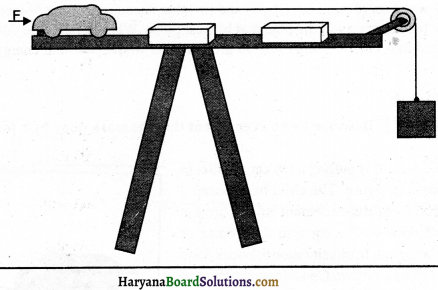Quick Review of the Chapter

1. What is required for doing a work?
(A) displacement in object
(B) force should be applied on the object
(C) both (A) and (B)
(D) no displacement in object
(C) both (A) and (B)

2. What type of energy is there inside a body kept at height?
(A) potential
(B) kinetic
(C) both (A) and (B)
(D) no relation with energy
(A) potential3. The device that converts the electrical energy In heat energy:
(A) Electrical iron
(B) Electrical bulb
(D) Electrical motor
(A) Electrical iron

4. Which type of energy is there in a flying bird?
(A) potential
(B) kinetic
(C) both (A) and (B)
(D) neither of the two
(C) both (A) and (B)

5. Rado transfer the electrical enegry in:
(A) light energy
(B) sound energy
(C) mechanical energy
(D) heat energy
(B) sound energy

6. Which formula is correct for work?
(A) W = $$\frac {F}{s}$$
(B) W = $$\frac {s}{F}$$
(C) W= Fs
(D) W = $$\frac {1}{Fs}$$
(C) W= Fs

7. The unit of force Is:
(A) Newton
(B) Newton metre
(C) Joule
(D) both (B) and (C)
(A) Newton

8. If 5n force acts on an object, then displacement is 2m, what will be the work done?
(A) lONm
(B) IOJ
(C) lOJm
(D) both(A)and(B)
(D) both (A) and (B)9. A coolie lifts an object of mass iS kg from the earth to 1.5 m keep it on his head. Calculate the
amount of work done by the coolie.
(A) 22.5J
(B) 2.25 J .
(C) 225J
(D) 225N
(C) 225 J

10. The potential energy of an object of mass 1kg kept at the height &f 2m will be:
(A) 9.8J
(B) 19.6J
(C) 29.4 J
(D) 39.2 J
(B) 19.6 J

11. Whose unit is Newton metre (Nm)?
(A) work
(B) force
(C) acceleration
(D) power
(A) work

12. How many watts are in 1 Kilowatt?
(A) 103
(B) 104
(C) 105
(D) 106
(A) 103

13. How many joules are in 1 Kilowatt?
(A) 36 lakhs
(B) 36 thousands
(C) 36 hundreds
(D) 36 crores
(A) 36 lakhs14. What is another name of newton metre?
(A) Erg
(B) Coulomb
(C) Joule
(D) Hertz
(C) Joule

15. ……….. is vector.
(A) potential energy
(B) kinetic energy
(C) work
(D) none of these
(D) none of these

16. A man stands for 10 minutes by carrying a weight of 20 kg the work done s ill be:
(A) 200joule
(B) onejoule
(C) zero
(D) 400joule
(C) zero

17. The unit of energy is:
(A) Nm
(B) Joule
(C) both (A) and (B)
(D) no unit
(C) both (A) and (B)

18. The example of kinetic energy is not:
(A) stone kept on mountain
(B) revolving wheel
(C) blowing air
(D) bullet shot out of a gun
(A) stone kept on mountain19. The example of potential energy is not :
(A) an arrow kept in the bow
(B) a stone on a mountain
(C) falling coconut
(D) a bullet in a gun
(C) falling coconut

20. An object of 10 kg mass is carried to a height of 6 m. Calculate the energy of the object. (g = 9.8 mc2)
(A) 98 J
(B) 58.8 J
(C) 588 J
(D) 5880 J
(C) 588 J

21. The law of conservation of mass is:
(A) all works require energy
(B) energy can be produced
(C) energy can be destroyed
(D) energy neither can be produced nor destroyed
(D) energy neither can be produced nor destroyed

22. Which statement is not correct?
(A) Potential energy + kinetic energy constant
(B) Kinetic energy + potential energy = mechanical energy
(C) As much decrease is there in potential energy at a point so is in kinetic energy
(D) As much decrease is there in potential energy so much increase takes place in kinetic energy.
(C) As much decrease is there in potential energy at a point so is in kinetic energy

23. Which formula is correct?
(A) P = W x t
(B) P = $$\frac {W}{t}$$
(C) P = $$\frac {t}{W}$$
(D) P = $$\frac{1}{W \times t}$$
(B) P = $$\frac {W}{t}$$

24. What is the unit of power?
(A) Joule
(B) Nm
(C) erg
(D) Watt
(D) Watt25. How much energy will be consumed by a person of 600 newton weight for climbing a height of 10m?
(A) 60 joule
(B) 600 jouie
(C) ó000joule
(D) 588 joule
(C) 6000 joule

26. When will be the minimum amount of potential energy in your body ? When you:
(A) are standing
(B) are sitting in a chair
(C) ire lying on the earth
(D) are sitting on the earth
(C) are lying on the earth

27. When ¡s work not done?
(A) on fixing a nail in a wood
(B) on sliding a box on the floor
(C) when there is no constituent of parallel force in the direction of motion
(D) a weight hanging on a peg
(C) when there is no constituent of parallel force in the direction of motion

28.
10 seconds are taken to fetch a bucket of 5 kg mass from a well of 10 m depth. The power used in the power will be:
(A) 5ONm
(B) 50)
(C) 50 W
(D) 500 W
(C) 50 W

29. How much energy will be consumed by 5 fans of 100 watt each in 4 hours?
(A) 20 kwh
(B) 10 kwh
(C) 2 kwh
(D) 1 kwh
(C)2 kwh

30. The rate of doing work is called:
(A) Power
(B) Force
(C) Energy
(D) None of these(C) $$\frac {1}{2}$$ mυ2
(C) $$\frac {1}{2}$$ mυ2